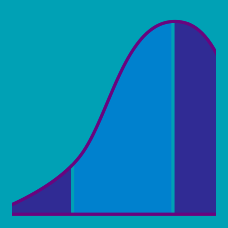Probability

# Continuous Random Variables - Probability Density Function (PDF)

If the probability density function of a continuous random variable $X\in[-1,7]$ is given by $f(x)=a(x+1)(x-7),$ what is $P(0\le X\le4)?$

The probability density function of a continuous random variable $X$ that ranges from 1 to $e^{2}$ is given by $f(x)=\frac{1}{2x}.$ Find the value of $k$ such that $P(X>k)=\frac{1}{10}.$

If the probability density function of a continuous random variable $X$ is given by $f(x)=\begin{cases} ax^2\ &(0\le x\le1) \\-\frac{a}{9}(x-1)+a\ &(1 what is the value of $a?$

If the probability density function of a continuous random variable $X\in[0,\frac{\pi}{16}]$ is given by $f(x)=a\sin8x,$ what is the variance of $X$ divided by $\pi-3 ?$

If the probability density function of a continuous random variable $X$ is given by $f(x)=11x^{10}\ (0\le x\le1),$ what is $E[X]?$

×